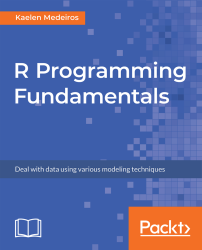•#### R Programming Fundamentals#### Overview of this book

R Programming Fundamentals, focused on R and the R ecosystem, introduces you to the tools for working with data. You’ll start by understanding how to set up R and RStudio, followed by exploring R packages, functions, data structures, control flow, and loops. Once you have grasped the basics, you’ll move on to studying data visualization and graphics. You’ll learn how to build statistical and advanced plots using the powerful ggplot2 library. In addition to this, you’ll discover data management concepts such as factoring, pivoting, aggregating, merging, and dealing with missing values. By the end of this book, you’ll have completed an entire data science project of your own for your portfolio or blog.
PrefaceFree Chapter
Introduction to RData Visualization and GraphicsData ManagementSolutionsOther Books You May Enjoy# Introduction to R

One tool that statisticians—and now data scientists as well—often use for data cleaning, analysis, and reporting is the R programming language.

In this chapter, we'll begin by looking at the basics of using R as a programming language and as a statistical analysis tool, and we'll also install a few useful R packages that we will continue to use throughout the book.

By the end of this chapter, you will be able to:

• Install R packages for use throughout the book
• Use R as a calculator for basic arithmetic
• Utilize different data structures
• Control program flow by writing if-else, for, and while loops
• Import and export data to and from CSV, Excel, and SQL